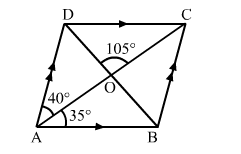# In the adjoining figure, ABCD is a parallelogram in which ∠BAO = 35°, ∠DAO = 40° and ∠COD = 105°.

Question:

In the adjoining figure, ABCD is a parallelogram in which BAO = 35°, ∠DAO = 40° and ∠COD = 105°. Calculate (i) ∠ABO, (ii) ∠ODC, (iii) ∠ACB, (iv) ∠CBD.Solution:

ABCD is a parallelogram.
∴ AB ∣∣​ DC and BC ​∣∣​ AD

(i) In ∆AOB, ∠BAO = 35°, ​∠AOB = ∠COD = 105°  (Vertically opposite angels)
∴ ​∠ABO = 180o − (35o + 105o) = 40o

(ii)∠ODC and ∠ABO are alternate interior angles.
∴ ∠ODC = ∠ABO = 40o

(iii) ∠ACB = ∠​CAD = 40o                             (Alternate interior angles)

(iv) ∠CBD = ABC  ABD            ...(i)